# Painlevé equation

(diff) ← Older revision | Latest revision (diff) | Newer revision → (diff)

The common name for a group of six special ordinary differential equations of the type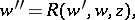whereis a rational function in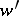and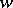and an analytic function in. Any such equation having only fixed singular points (cf. Movable singular point) can be reduced to one out of 50 canonical equations. These include linear equations, Riccati equations (cf. Riccati equation) and other well-known equations, as well as the six other equations known as the Painlevé equations, and having solutions in the form of transcendental Painlevé functions, which are special functions that do not reduce to other known ones. Arranged in the generally-accepted order, the Painlevé equations have the following form (are constants):

1)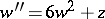;

2)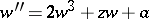;

3),;

4)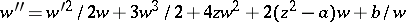;

5)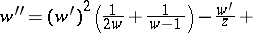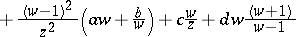;

6)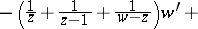.

These results were first obtained in researches by P. Painlevé , . These were continued, refined and supplemented by B. Gambier .